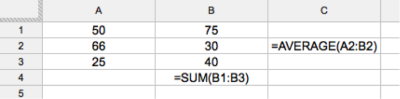In the Spreadsheets portion of the TSA, you will be required to use Google Drive to produce a document similar to the examples shown on this page.

If you have never used Google Forms or Spreadsheets before, there are excellent tutorials created by Google that will teach you the basics of both.

It is recommended that you learn Google Forms first. When you create a Google Form, you are able to connect it to a Google Spreadsheet to record the information submitted to the Form.### Creating basic formulas

Here are some basic formulas entered into a Google Spreadsheet. Formulas begin with an equals sign (=). They can be as simple as an arithmetic expression (6/3 in cell C4) and can reference other cells (as in cell A4).Here is what the final spreadsheet would look like.Use Spreadsheet Assistance  to search using key words and phrases such as formulas .

There are key words, called functions , that can be used in formulas for more complex tasks. In the example below, notice the SUM function in cell B4. It will add up the numbers in the range B1:B3 (B1+B2+B3). The function AVERAGEdoes the same thing, but divides the total by the number of data-filled cells in the range.Here is what the final spreadsheet would look like:For a list of possible formulas, use the  Spreadsheet Formula List . Try searching these words :

• average
• sum
• multiply
• subtract

### Inserting a chart

Charts are sometimes called graphs . They can be bar charts, line charts, pie charts, etc. Make sure all of the information you want on your chart, including words, is selected in the range.

Using the Create and Edit Charts you can find anything you may need to know about charts. Or you can search using any of these key phrases:

• Create a Chart
• Chart Types